## Examples

Example 3.1.2   Find the area enclosed by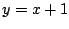,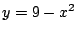,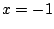,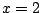.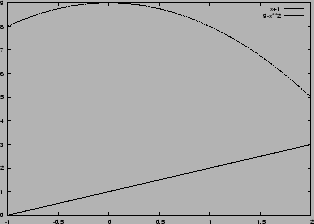Area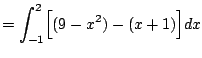We have reduced the problem to a computation: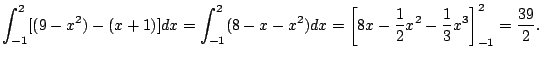The above example illustrates the simplest case. In practice more interesting situations often arise. The next example illustrates finding the boundary points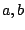when they are not explicitly given.

Example 3.1.3   Find area enclosed by the two parabolas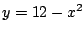and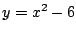.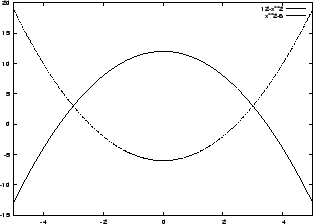Problem: We didn't tell you what the boundary pointsare. We have to figure that out. How? We must find exactly where the two curves intersect, by setting the two curves equal and finding the solution. We have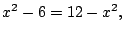so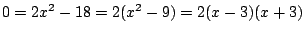, hence the intersect points are at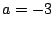and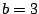. We thus find the area by computing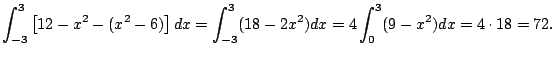Example 3.1.4   A common way in which you might be tested to see if you really understand what is going on, is to be asked to find the area between two graphs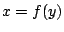and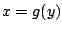. If the two graphs are vertical, subtract off the right-most curve. Or, just switch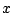and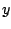'' everywhere (i.e., reflect about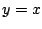). The area is unchanged.

Example 3.1.5   Find the area (not signed area!) enclosed by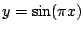,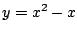, and.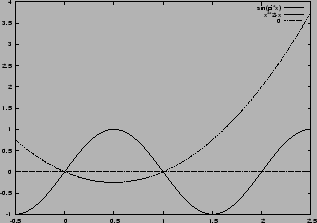Write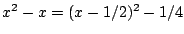, so that we can obtain the graph of the parabola by shifting the standard graph. The area comes in two pieces, and the upper and lower curve switch in the middle. Technically, what we're doing is integrating the absolute value of the difference. The area is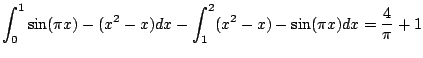Something to take away from this is that in order to solve this sort of problem, you need some facility with graphing functions. If you aren't comfortable with this, review.

William Stein 2006-03-15